# NATA Aesthetic Sensitivity Test Sample Paper 7

## 39 Questions MCQ Test NATA - Sample Paper & Mock Tests | NATA Aesthetic Sensitivity Test Sample Paper 7

Description
Attempt NATA Aesthetic Sensitivity Test Sample Paper 7 | 39 questions in 78 minutes | Mock test for JEE preparation | Free important questions MCQ to study NATA - Sample Paper & Mock Tests for JEE Exam | Download free PDF with solutions
QUESTION: 1

Solution:
QUESTION: 2

### What time should the bottom clock show?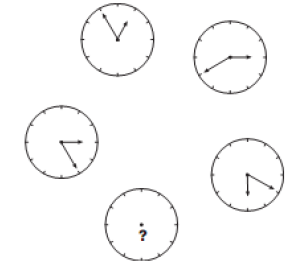Solution:
Explanation Starting with the top clock and moving anti-clockwise around the others, the hour hand moves back 1 hour, then 2, then 3 etc, while the minute hand moves forward 10 minutes each time.
QUESTION: 3

### There is some relationship between diagrams A & B. The same relationship persists between C & D. Find the right diagrams for D from the alternatives.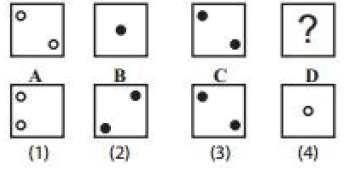Solution:
QUESTION: 4

Choose the figure which is different: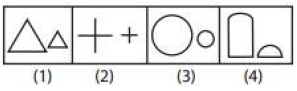Solution:
QUESTION: 5

Light year is a unit of

Solution:
QUESTION: 6

Identify the given image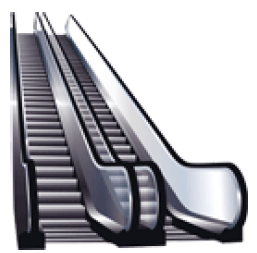Solution:
QUESTION: 7

Identify the building given below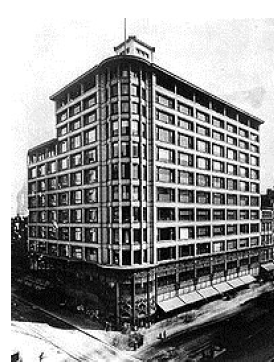Solution:
QUESTION: 8

Q is as much younger than R as he is older than T. If the sum of the ages of R and T is 50 years, what is definitely the difference between R and Q's age?

Solution:
QUESTION: 9

P, Q and R can do a work in 20, 30 and 60 days respectively. How many days does it need to complete the work if P does the work and he is assisted by Q and R on every third day?

Solution:
Amount of work P can do in 1 day = 1/20

Amount of work Q can do in 1 day = 1/30

Amount of work R can do in 1 day = 1/60

P is working alone and every third day Q and R is helping him

Work completed in every three days = 2 x (1/20) + (1/20 + 1/30 + 1/60) = 1/5

So work completed in 15 days = 5 x 1/5 = 1

Ie, the work will be done in 15 days
QUESTION: 10

A man has some hens and cows. If the number of heads be 48 and the number of feet equals 140, then the number of hens will be

Solution:
QUESTION: 11

Who is this architect?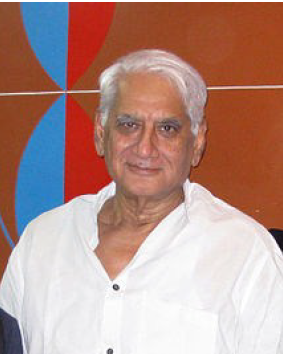Solution:
QUESTION: 12

Identify the building given below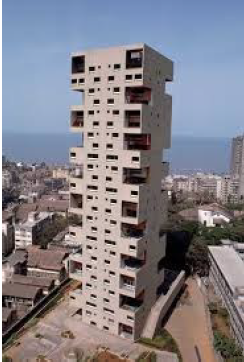Solution:
QUESTION: 13

Find the odd one out

Solution:
QUESTION: 14

Identify the image